# Python基础知识（3） | “朝闻道”知识分享大赛

## 五. Python函数

### 1. 函数介绍

(1) 函数：是组织好的，可重复使用的，用来使用特定功能的代码段。

• 将功能封装在函数内，可供随时随地重复利用
• 提高代码的复用性，减少重复代码，提高开发效率
``````# 需求：统计字符串的长度，且不使用内置函数len()
str1="itheima"
str2="itcast"
str3="python"
def my_len(data):
count=0
for i in data:
count+=1
print(f"字符串{data}的长度是{count}")
my_len(str1)
my_len(str2)
my_len(str3)

``````

### 2. 函数的定义

(1) 语法：

def 函数名(传入参数)：

​ 函数体

​ return 返回值

(2) 函数的调用： 函数名(传入参数)

``````def say_hi():
print("hello")

say_hi()

hello
``````

(3) 注意：

• 执行顺序时从上向下，函数必须先定义后使用。
• 参数如不需要，可以省略；返回值如不需要，可以省略。

### 3. 函数的参数

(1) 传入参数的功能是：在函数进行计算的时候，接受外部(调用时)提供的数据。

``````# 定义相加的函数
result=x+y
print(f"{x}+{y}的计算结果是:{result}")
# 调用函数，传入被计算的2个数字

5+6的计算结果是:11
``````
• 函数定义中，提供的x和y，称为形式参数(形参)，表示函数声明将要使用2个参数：参数之间用逗号隔开。
• 函数调用中，提供的5和6，称为实际参数(实参)，表示函数执行时真正使用的参数值：传入的时候，按照顺序传入数据，使用逗号隔开。
• 传入参数的数量是不受限制的，传入参数时，要和形式参数一一对应，逗号隔开。

### 4. 函数的返回值

(1) 语法：

def 函数名(传入参数)：

​ 函数体

​ return 返回值

``````# 定义一个函数，完成两数相加的功能
result=a+b
return  result
# 返回结果后，还想输出一句话 return后面的代码都不会执行了
print("我完事了")
# 函数的返回值，可以通过变量去接收
print(r)

4
``````
• 使用return关键字来返回结果。
• 函数体在遇到return后就结束了，所以写在return后的代码不会执行。

(2) 函数返回值之None类型

• 用在函数无返回值上：无返回值的函数，实际上就是返回了一个特殊的字面量None，其类型是：<class 'NoneType'>，也就是返回了空的意思。
• 用在if判断上：在if判断中，None等同于False；一般用于在函数中主动返回None，配合if判断做相关处理。
• 用于声明无内容的变量上：定义变量，但暂时不需要变量有具体值，可以用None来代替。name = None
``````# 返回None类型
def say_hi():
print("Hello")

res=say_hi()
print(res)
print(type(res))

Hello
None
<class 'NoneType'>
``````
``````# None用于if判断
def check_age(age):
if age>18:
return "SUCCESS"
else:
return None
result=check_age(16)
if not result:
# 进入if表示result是None值，也就是False
print("未成年，不可以进入")

``````

### 5. 函数说明文档

(1) 给函数添加说明文档，辅助理解函数的作用。

(2) 语法：

def func(x,y):

​ """

​ 函数说明

​ :param x:形参x的说明

​ :param y:形参y的说明

​ :return:返回值的说明

​ """

​ 函数体

​ return 返回值

``````# 定义函数，进行文档说明

"""
# 自动补全
:param x: 形参x表示相加的其中一个数字
:param y: 形参y表示相加的另一个数字
:return: 返回值是两数相加的结果
"""
result=x+y
print(f"两数相加的结果是:{result}")
return  result
``````

(3) 在PyCharm编写代码是，可以通过鼠标悬停，查看调用函数的说明文档。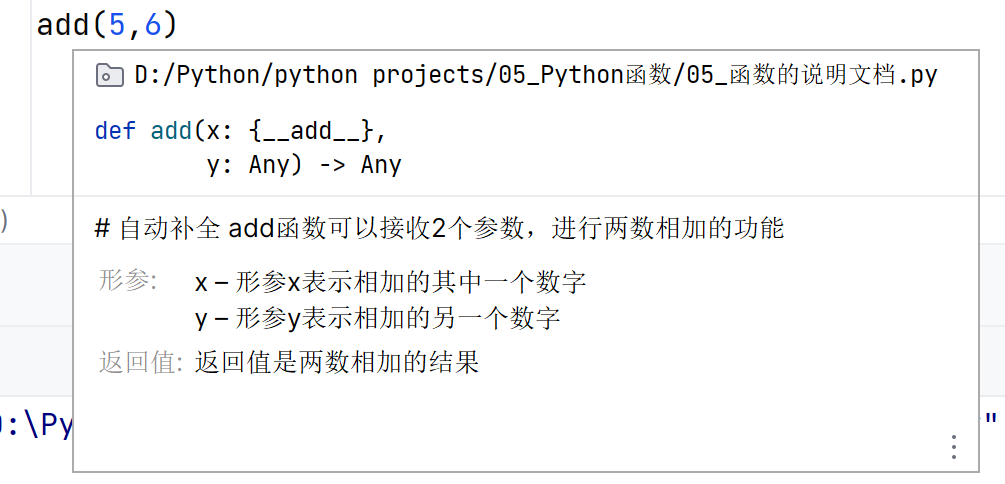### 6. 函数的嵌套调用

(1) 定义：函数的嵌套调用指的是一个函数里面又调用了另一个函数。

``````# 定义函数func_b
def func_b():
print("----2----")

# 定义函数func_a，并在内部调用func_b
def func_a():
print("----1----")
# 嵌套调用func_b
func_b()
print("----3----")

func_a()

----1----
----2----
----3----
``````

(2) 如果函数a中，调用了另一个函数b，那么先把函数b中的任务都执行完毕后才会回到上次函数a执行的位置，完成后，继续执行函a剩余的内容。

### 7. 变量的作用域

(1) 变量作用域指的是变量的作用范围(变量在哪里可用，在哪里不可用)，主要分为两类：局部变量和全局变量。

(2) 局部变量：定义在函数体内部的变量，即只在函数体内部生效。作用：在函数体内部，在函数运行时临时保存数据，当函数调用完成之后，立刻销毁了局部变量。

``````# 演示局部变量
def test_c():
num=100
print(num)

test_a()
# 出了函数体，局部变量就无法使用了
# print(num)
``````

(3) 全局变量：指的是在函数体内、外都能生效的变量。如果有一个数据，在函数a和函数b中都要使用，就可以将这个数据存储在一个全局变量中。定义这个变量在函数的外部。

``````# 演示全局变量
num=200
def test_a():
print(f"test_a:{num}")

def test_b():
print(f"test_b:{num}")

test_a()
test_b()
print(num)

test_a:200
test_b:200
200
``````

(4) global关键字：可以在函数内部声明变量为全局变量。

``````# 使用global关键字，在函数内声明变量为全局变量
num=200
def test_a():
print(f"test_a:{num}")

def test_b():
global num
num=500
print(f"test_b:{num}")

test_a()
test_b()
print(num)  # 输出的是500
``````

## 六. Python数据容器

### 1. 数据容器入门

(1) 数据容器：一个容器可以容纳多份数据。

(2) 定义：一种可以容纳多份数据的数据类型，容纳的每一份数据称之为1个元素，每一个元素，可以是任意类型的数据，如字符串、数字、布尔等。

(3) 数据容器根据特点的不同，如：

• 是否支持重复元素
• 是否可以修改
• 是否有序，等

### 2. 数据容器

#### 2.1 列表 list [ ]

(1) 基本语法：

• 字面量：[元素1，元素2，元素3，元素4，.......]
• 定义变量： 变量名称 = [元素1，元素2，元素3，元素4，.......]
• 定义空列表：变量名称 = [ ] ； 变量名称 = list ( )

``````# 定义一个列表list
name_list=["itheima","itcast","python"]
print(name_list)
print(type(name_list))

my_list=["itheima",666,True]
print(my_list)
print(type(my_list))

['itheima', 'itcast', 'python']
<class 'list'>
['itheima', 666, True]
<class 'list'>
``````

(2) 注意：

• 列表可以一次存储多个数据，且可以为不同的数据类型，支持嵌套。
``````# 定义一个嵌套的列表
my_list2=[[1,2,3],[4,5,6]]
print(my_list2)
print(type(my_list2))

[[1, 2, 3], [4, 5, 6]]
<class 'list'>
``````

(3) 列表的下标(索引)：使用[ ]

• 正向：列表中的每一个元素，都有其位置下表索引，从前向后的方向，从0开始，依次递增。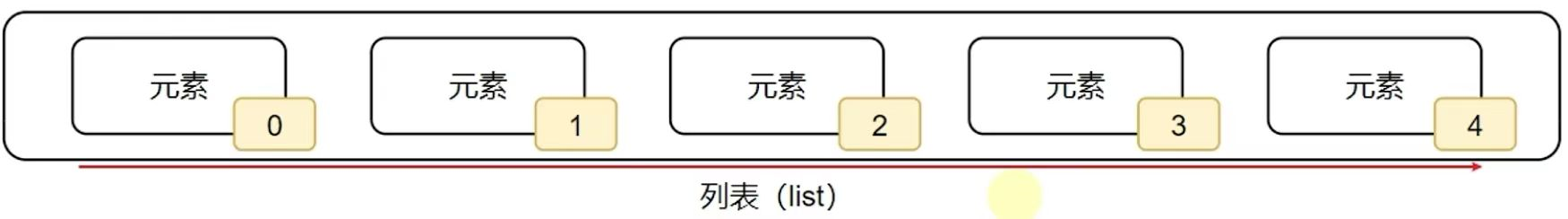• 反向：反向索引，也就是从后向前，从-1开始，依次递减(-1，-2，-3，........)。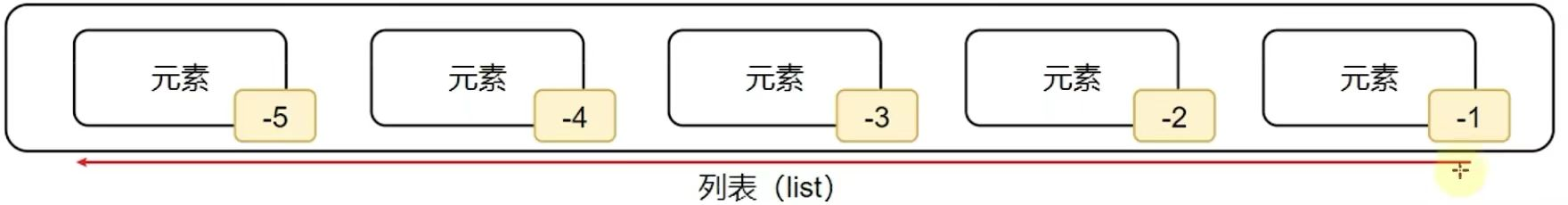• 嵌套列表的索引：同样支持下标索引。列表名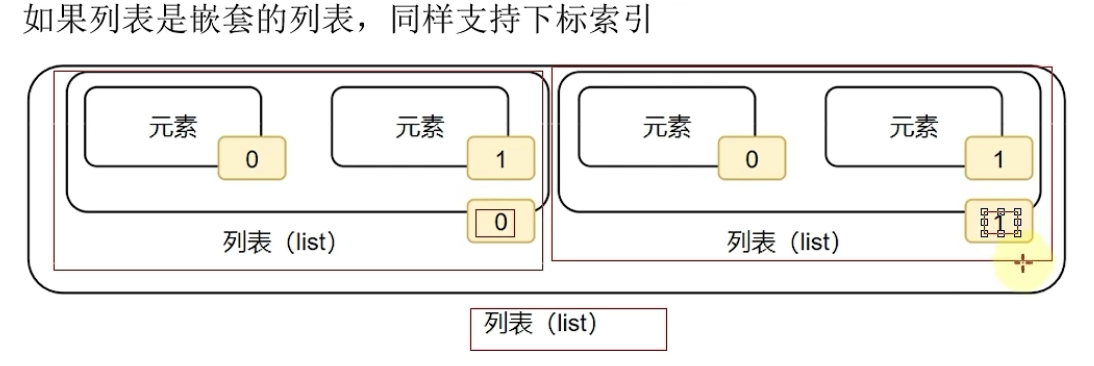``````# 列表[下表索引]，从前向后，从0开始，每次+1   从后向前，从-1开始，每次-1
print(my_list)  # Tom
print(my_list)  # Lily
print(my_list)  # Rose
# 错误示范：通过下标索引取出数据，一定不要超出范围
# print(my_list)  # 超出范围，数组越界

# 通过下标索引取出数据(倒序取出)
print(my_list[-1]) # Rose
print(my_list[-2]) # Lily
print(my_list[-3]) # Tom

# 取出嵌套列表的元素
my_list = [[1,2,3],[4,5,6]]
print(my_list) # 5
``````

(4) 列表的常用操作(方法)：

• 方法的使用: student = Student() num=student.add(1,2)

① 列表的查询功能(方法)：

• 查找某元素的下标：查找指定元素在列表的下标，如果找不到，报错ValueError。

—— **语法：列表.index(元素)**。index就是列表对象(变量)内置的方法(函数)。

• 统计列表内，有多少元素：

—— **语法：len(列表)**。

② 列表的修改功能(方法)：

• 修改特定位置(索引)的元素值：直接对指定下标(正向、反向下标均可)的值进行重新赋值(修改)。

—— 语法：列表[下标] = 值

• 插入元素：在指定的下标位置，插入指定的元素。

—— **语法：列表.insert(下标，元素)**。

• 追加元素：将指定元素，追加到列表的尾部。

—— **语法1：列表.append(元素)**。

—— **语法2：列表.extend(其他数据容器)**。将其它数据容器的内容取出，依次追加到列表尾部。

• 删除元素：

—— **语法1：del 列表[下标]**。

—— **语法2：列表.pop(下标)**。

• 删除某元素在列表中的第一个匹配项：

—— **语法：列表.remove(元素)**。

• 清空列表内容：

—— **语法：列表.clear( )**。

• 统计某元素在列表内的数量:

—— **语法：列表.count(元素)**。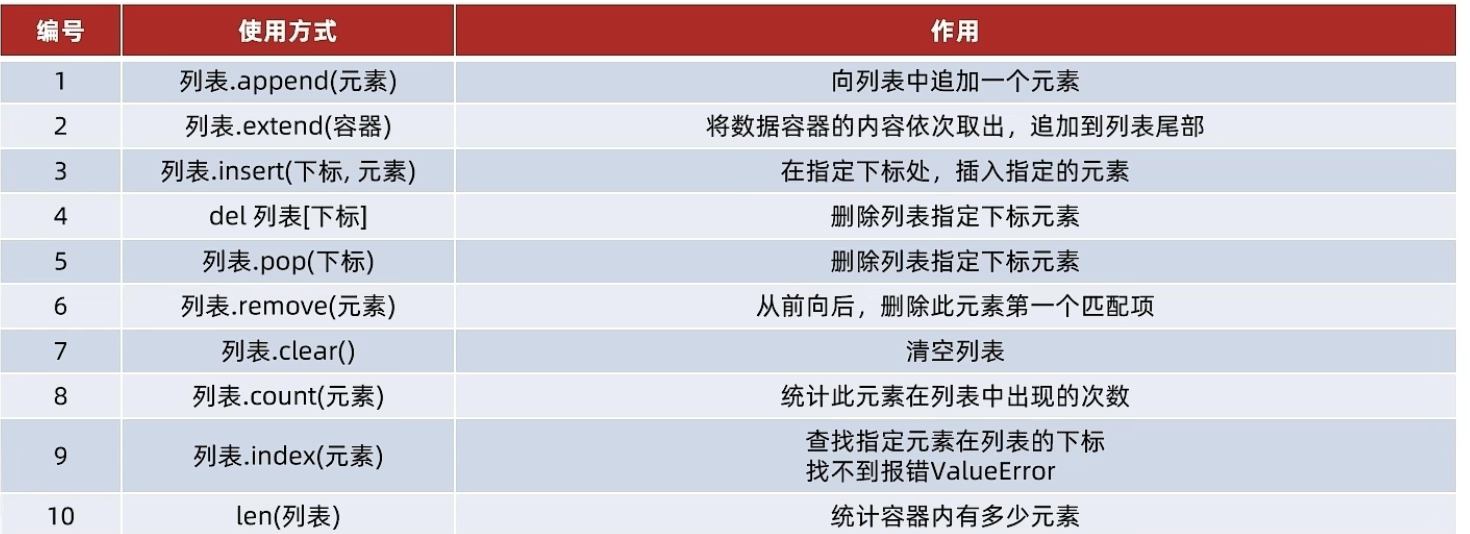(5) 列表的特点：

• 可以容纳多个元素。
• 可以容纳不同类型的元素(混装)。
• 数据是有序存储的(有下标序号)。
• 允许重复数据存在。
• 可以修改(增加或者删除等)。

(6) 列表的遍历：

① while循环：定义一个变量表示下标，从0开始，循环条件为下标值<列表的元素数量，使用列表[下标]的方式取出。

``````def list_while_func():
"""
使用while循环遍历列表的演示函数
:return: None
"""
mylist=["传智教育","黑马程序员","Python"]
index=0
while index<len(mylist):
element=mylist[index]
print(f"列表的元素{index+1}:{element}")
index+=1

list_while_func()
``````

② for循环：从容器内，依次取出元素并赋值到临时变量上。在每一次的循环中，我们可以对临时变量(元素)进行处理。

``````def list_for_func():
"""
使用for循环遍历列表的演示函数
:return: None
"""
mylist=[1,2,3,4,5]
for element in mylist:
print(f"列表的元素有:{element}")

list_for_func()
``````

③ 两者对比：

• 在循环控制上：
• while循环可以自定循环条件，并自行控制
• for循环不可以自定循环条件，只能一个个从容器内取出数据
• 在无限循环上：
• while循环可以通过条件控制做到无限循环
• for循环理论上不可以，因为被遍历的容器容量不是无限的
• 在使用场景上：
• while循环适用于任何想要循环的场景
• for循环适用于，遍历数据容器的场景或者简单的固定次数循环场景

#### 2.2 元组 tuple ( )

(1) 列表是可以修改的，如果想要传递的信息不被篡改，就需要元组。

`````` 元组一旦定义完成，就不可修改。
``````

(2) 基本语法：定义元组使用小括号，且使用逗号隔开各个数据，数据可以是不同类型的

• 元组字面量：(元素，元素，元素，.........)
• 元组变量：变量名称 = (元素，元素，元素，.........)
• 空元祖：变量名称 = ( ) ; 方式1 变量名称 = tuple ( ) ;方式2，实际上是得到元组的类对象
``````t1=(1,"Hello",True)
t2=()
t3=tuple()
print(f"t1的类型是:{type(t1)}，内容是:{t1}")
print(f"t2的类型是:{type(t2)}，内容是:{t2}")
print(f"t3的类型是:{type(t3)}，内容是:{t3}")
``````
• 当元组只有一个数据，这个数据后面要添加逗号
``````# 定义单个元素的元组
t4=("hello",)
print(f"t4的类型是:{type(t4)}，内容是:{t4}")

t4的类型是:<class 'tuple'>，内容是:('hello',)
``````
• 元组的嵌套：
``````# 元组的嵌套
t5=((1,2,3),(4,5,6))
print(f"t5的类型是:{type(t5)}，内容是:{t5}")

t5的类型是:<class 'tuple'>，内容是:((1, 2, 3), (4, 5, 6))
``````

(3) 元组的下标(索引)：同列表list一样

(4) 元组的相关操作：

• index( )：查找某个数据，如果数据存在返回对应的下标，否则报错。
• count( )：统计某个数据在当前元组出现的次数。
• len(元组)：统计元组内的元素个数。
• 元组不可修改，但是如果里面有列表是可以的。
``````# 元组内容不可以修改，但是元组中的列表可以
t6=(1,2,["itheima","itcast"])
t6="tef"
t6="redf"
print(f"t6的内容是:{t6}")   # t6的内容是:(1, 2, ['tef', 'redf'])
``````

(5) 元组的特点：

• 可以容纳多个数据。
• 可以容纳不同类型的数据(混装)。
• 数据是有序存储的(下标索引)。
• 允许重复数据存在。
• 不可以修改(增加或者删除元素等)。
• 支持for循环。

#### 2.3 字符串 str " "

(1) 定义：字符串是字符的容器，一个字符串可以存放任意数量的字符。每一个字符就是一个元素，每一字符也有下标索引。

(2) 字符串的下标(索引)：和其他容器一样：列表、元组一样，字符串也可以通过下标进行访问。

• 从前向后，下标从0开始
• 从后向前，下标从-1开始

(3) 同元组一样，字符串是一个：无法修改的容器。字符串的相关操作：

• 查找特点字符串的下标索引值：字符串.index(字符串)。
• 字符串的替换：字符串.repalce(字符串1，字符串2)。—— 将字符串内的全部内容字符串1替换为字符串2。不是修改字符串本身，而是得到了一个新字符串，实质上相当于返回了一个新的字符串。
``````# replace方法
new_my_str=my_str.replace("it","程序")
print(f"将字符串{my_str}，进行替换之后得到:{new_my_str}")

``````
• 字符串的分割：字符串.split(分隔符字符串)。 —— 按照指定的分隔符字符串，将字符串划分为多个字符串，并存入列表对象中。字符串本身不变，而是得到了一个列表对象。
``````# split方法
my_str="hello python itheima and itcast"
my_str_list=my_str.split(" ")  #按照空格划分,返回一个列表
print(f"将字符串{my_str}进行分割后,得到:{my_str_list},类型是:{type(my_str_list)}")

``````
• 字符串的规整操作：—— 会得到一个新的字符串
• 去除前后空格：字符串.strip()。
• 去除前后指定字符串：字符串.strip(字符串)。
``````# strip方法
my_str="  itheima and itcast  "
new_my_str=my_str.strip() #不传入参数，去除首尾空格
print(f"字符串{my_str}进行strip后，结果是:{new_my_str}")

my_str="12itheima and itcast21"
new_my_str=my_str.strip("12")
print(f"字符串{my_str}进行strip(‘12’)后，结果是:{new_my_str}")
# 并不是完全按照12来的，只要满足其中任意均可去除，实质上是按照每个字符来去除

``````
• 统计字符串内某字符串的出现次数：字符串.count(字符串)。
• 统计字符串的字符个数(包括空格也是一个字符)：len(字符串)。

(4) 字符串的特点：

• 只可以存储字符串
• 长度任意(取决于内存大小)
• 支持下标索引
• 允许重复字符串存在
• 不可以修改(增加或者删除元素等)
• 支持for循环

(5) 字符串大小比较：

• 字符串是按位比较，也就是一位位进行对比，只要有一位大，那么整体就大。
• 通过ASCII码表，确定字符对应的码值数字来确定大小。

#### 2.4 集合 set { }

(1) 集合最主要的特点就是：不支持元素的重复(自带去重功能)，并且内容无序。

(2) 基本语法：和列表、元组、字符串等定义基本相同：

• 定义集合字面量：{元素1，元素2，元素3，..........，元素n}
• 定义集合变量：变量名称 = {元素1，元素2，元素3，..........，元素n}
• 定义空集合：变量名称 = set( )
``````# 定义集合
my_set={"传智教育","黑马程序员","itheima","传智教育","黑马程序员","itheima","传智教育","黑马程序员","itheima"}
my_empty_set=set()  #定义空集合
print(f"my_set的内容是:{my_set},类型是:{type(my_set)}")  # 不能重复，内容无序
print(f"my_empty_set的内容是:{my_empty_set},类型是:{type(my_empty_set)}")

my_set的内容是:{'itheima', '传智教育', '黑马程序员'},类型是:<class 'set'>
my_empty_set的内容是:set(),类型是:<class 'set'>
``````

(3) 因为集合是无序的，所以集合不支持：下标索引访问。但是集合和列表一样，是允许修改的。

• 集合的常用操作 —— 修改

• 移除元素：集合.remove(元素)。将指定元素，从集合内移除。集合本身被修改，移除了元素。
• 从集合中随机取出元素：集合.pop()。从集合中随机取出一个元素。会得到一个元素的结果，同时集合本身被修改，元素被移除。
• 清空集合：集合.clear()。
• 取出2个集合的差集：集合1.difference(集合2)。取出集合1和集合2的差集(集合1有而集合2没有的)。得到一个新集合，集合1和集合2不变。
``````# 取出2个集合的差集
set1={1,2,3}
set2={1,5,6}
set3=set1.difference(set2)
print(set3)  #{2,3} 集合1有而集合2没有的
``````
• 消除2个集合的差集：集合1.difference_update(集合2)。对比集合1和集合2，在集合1内，删除和集合2相同的元素。集合1被修改，集合2不变。
``````# 消除2个集合的差集
set1.difference_update(set2)
print(set1)  # {2,3} 没有了1,删除了相同的
print(set2)  # {1, 5, 6} 集合2不变
``````
• 2个集合合并：集合1.union(集合2)。将集合1和集合2合成新集合，有相同的只有一个。得到新集合，集合1和集合2不变，也不能保证新集合的顺序。
``````# 2个集合合并
set1={1,2,3}
set2={1,5,6}
set3=set1.union(set2)
print(set3) # {1, 2, 3, 5, 6}  去重，只保留一个1
``````
• 统计集合元素的数量：len(集合)。得到一个整数表示集合的元素数量。

(4) 集合的特点：

• 可以容纳多个数据
• 可以容纳不同类型的数据(混装)
• 数据是无序存储的(不支持下标索引)
• 不允许重复数据存在
• 可以修改(增加或删除元素等)
• 支持for循环

#### 2.5 字典/映射 dict

(1) 使用字典，实现用Key取出Value的操作。定义：同样使用{}，不过存储的是一个个的:键值对。

(2) 基本语法：

• 定义字典字面量：{key1:value1,key2:value2,.............,keyn:valuen}
• 定义字典变量：my_dict(变量名)={key1:value1,key2:value2,.............,keyn:valuen}
• 定义空字典：my_dict={ } ; my_dict=dict( )

(3) 字典数据的获取：字典同集合一样，不可以使用下标索引，但是字典可以通过Key值来取得对应的Value。

• 字典[Key]可以取到对应Value
``````# 从字典中基于Key获取Value
my_dict1={"王力宏":99,"周杰伦":88,"林俊杰":77}
score1=my_dict1["周杰伦"]
print(f"周杰伦的分数是:{score1}")

``````

(4) 字典的嵌套：字典的Key和Value可以是任意数据类型(Key不可以为字典)。这就表明，字典是可以嵌套的。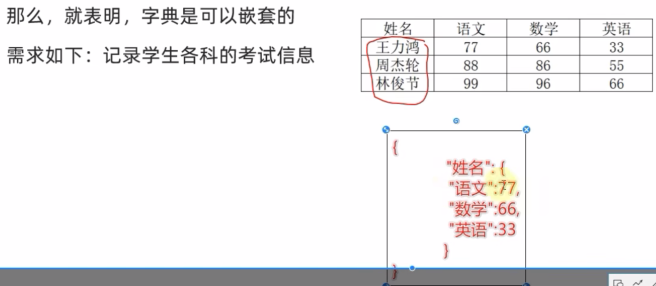``````# 定义嵌套字典
stu_score_dict = {
"王力宏":{
"语文":77,
"数学":66,
"英语":33
},"周杰伦":{
"语文":88,
"数学":86,
"英语":55
},"林俊杰":{
"语文":99,
"数学":96,
"英语":66
}
}
print(f"学生的考试信息是:{stu_score_dict}")

# 从嵌套字典中获取数据
# 查看周杰伦的语文成绩
zhou_chinese=stu_score_dict["周杰伦"]["语文"]
print(f"周杰伦的语文成绩是:{zhou_chinese}")
# 查看林俊杰的英语成绩
lin_english=stu_score_dict["林俊杰"]["英语"]
print(f"林俊杰的英语成绩是:{lin_english}")

``````

(5) 字典的特点：

• 键值对的Key和Value可以是任意类型(Key不可为字典)
• 字典内Key不允许重复，重复添加等同于覆盖原有数据
• 字典不支持下标索引，而是通过Key检索Value
• 可以容纳多个数据
• 可以容纳不同类型的数据
• 每一份数据是Key-Value键值对
• 可以通过Key获取到Value，Key不可以重复(重复会覆盖)
• 不支持下标索引
• 可以修改(增加或者删除更新元素等)
• 支持for循环，不支持while循环

(6) 字典的常用操作：

• 新增元素：字典[Key]=Value。字典被修改，如果新增的元素不存在，直接新增了键值对。
• 更新元素：字典[Key]=Value。字典被修改，如果修改的元素存在，元素被更新，原有的数据被覆盖。
• 删除元素：字典.pop(Key)。获得指定的Key的Value，同时字典被修改，指定的Key的数据被删除。
• 清空元素：字典.clear()。
• 获取全部的key：字典.keys()。得到字典中全部的Key。
• 统计字典内的元素数量：len(字典)。
• 取键值对：字典.items()方法。返回所有的键值对。
• 取值：字典.values()方法。返回所有值信息。
• 获取指定“键”对应的“值”：字典.get(key,default)。键存在则返回相应值,否则返回默认值。
``````my_dict={"周杰伦":99,"林俊杰":88,"张学友":77}

# 新增元素
my_dict["张信哲"]=66
print(f"字典经过新增元素后，结果:{my_dict}")

# 更新元素
my_dict["周杰伦"]=33
print(f"字典经过更新元素后，结果:{my_dict}")

# 删除元素
zhou_score=my_dict.pop("周杰伦")
print(f"字典中被移除了一个元素，结果:{my_dict}，周杰伦的考试分数:{zhou_score}")

# 清空元素
my_dict.clear()
print(f"字典被清空了，内容是:{my_dict}")

# 获取全部的key
my_dict={"周杰伦":99,"林俊杰":88,"张学友":77}
keys=my_dict.keys()
print(f"字典的全部keys是:{keys}")

# 遍历字典

# 方式1：通过获取全部的key来完成遍历
for key in keys:
print(f"字典的key是:{key}   ",end="")
print(f"字典的value是:{my_dict[key]}")

# 方式2：直接对字典进行for循环
for key in my_dict:  # 每一次循环取出的就是key
print(f"字典的key是:{key}   ", end="")
print(f"字典的value是:{my_dict[key]}")

# 统计字典内的元素数量，len()函数
num=len(my_dict)
print(f"字典中的元素数量是:{num}")

``````

### 3. 数据容器(序列)的切片操作

#### 3.1 什么是序列

(1) 序列是指：内容连续、有序，可以使用下标索引的一类数据容器。列表、元组、字符串，均可以视为序列。

#### 3.2 序列的常用操作 —— 切片

(1) 切片：从一个序列中，取出一个子序列。

(2) 语法：序列[起始下标:结束下标:步长]。 —— 表示从序列中，从指定位置开始，依次取出元素，到指定位置结束，得到一个新序列。

• 起始下标表示从何处开始，可以留空，留空视作从头开始。
• 结束下标(不含)表示何处结束，可以留空，留空视作截取到结尾。
• 步长表示，依次取元素的间隔：
• 步长1表示，一个个取元素
• 步长2表示，每次跳过1个元素取
• 步长n表示，每次跳过n-1个元素取
• 步长为负数表示，反向取(注意，起始下标和结束下标也要反向标记)
``````# 对list切片，从1开始，4结束，步长1
my_list=[0,1,2,3,4,5,6]
result1=my_list[1:4:1]  # 步长默认是1，所以可以省略不写
print(f"结果1:{result1}")

# 对tuple进行切片，从头开始，到最后结束，步长1
my_tuple=(0,1,2,3,4,5,6)
result2=my_tuple[:]    #起始和结束不写，表示从头到尾，步长为1可以省略
print(f"结果2:{result2}")

# 对str进行切片，从头开始，到最后结束，步长为2
my_str="0123456"
result3=my_str[::2]
print(f"结果3:{result3}")

# 对str进行切片，从头开始，到最后结束，步长-1
my_str="0123456"
result4=my_str[::-1]  # 等同于将序列反转了
print(f"结果4:{result4}")

# 对列表进行切片，从3开始，到1结束，步长-1
my_list=[0,1,2,3,4,5,6]
result5=my_list[3:1:-1]
print(f"结果5:{result5}")

# 对元组进行切片，从头开始，到尾结束，步长-2
my_tuple=(0,1,2,3,4,5,6)
result6=my_tuple[::-2]
print(f"结果6:{result6}")

``````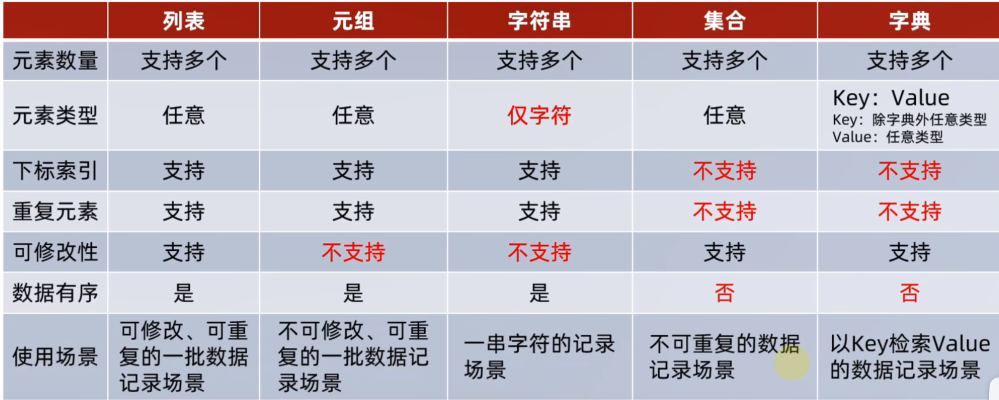### 4. 数据容器的通用操作

(1) 遍历

• 5类数据容器都支持for循环遍历
• 列表、元组、字符串支持while循环，集合、字典不支持(无法下标索引)

(2) 统计功能

• len(容器) —— 统计容器的元素个数
• max(容器) —— 统计容器的最大元素
• min(容器) —— 统计容器的最小元素

(3) 通用转换功能

• list(容器) —— 将给定容器转换为列表：字典转列表，将value全部舍弃，只留key。
• str(容器) —— 将给定容器转换为字符串
• tuple(容器) —— 将给定容器转换为元组
• set(容器) —— 将给定容器转换为集合

(4) 通用排序功能

• sorted(容器，reserve=True)。将给定容器进行排序。默认为False，从小到大升序排列。reverse=True表示降序
• 排序的结果会统统变成列表项。
``````# 进行容器排序
my_list=[3,1,2,5,4]
my_tuple=(3,1,2,5,4)
my_str="bdcefga"
my_set={3,1,2,5,4}
my_dict={"key3":1,"key1":2,"key2":3,"key5":4,"key4":5}
print(f"列表对象的排序结果:{sorted(my_list,reverse=True)}")
print(f"元组对象的排序结果:{sorted(my_tuple)}")
print(f"字符串对象的排序结果:{sorted(my_str)}")
print(f"集合对象的排序结果:{sorted(my_set)}")
print(f"字典对象的排序结果:{sorted(my_dict)}")

``````
...全文
24 回复 打赏 收藏 转发到动态 举报蓝桥杯思路分析.docx算法组-python中函数的部分基础知识总结|“朝闻知识分享大赛
python中函数的部分基础知识总结蓝桥杯python知识总结(详细)全国青少年信息素养大赛Python编程挑战赛初赛试题说明大数协Python训练营Python基础知识学习笔记

833发帖与我相关我的任务• 近7日
• 近30日
• 至今

CSDN高校俱乐部是给大家提供技术分享交流的平台，会不定期的给大家分享CSDN方面的相关比赛以及活动或实习报名链接，希望大家一起努力加油！共同建设中南民族大学良好的技术知识分享社区。

1.社区成员不得在社区发布违反社会主义核心价值观的言论。

2.社区成员不得在社区内谈及政治敏感话题。

3.该社区为知识分享的平台，可以相互探讨、交流学习经验，尽量不在社区谈论其他无关话题。